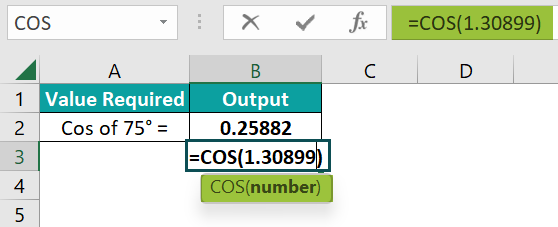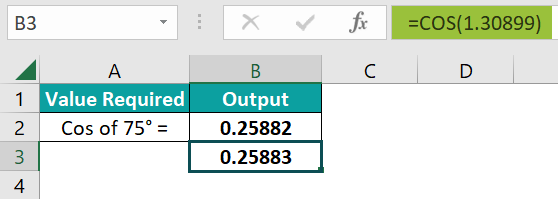# COS Excel FunctionArticle byExcelMojo Team## What Is COS Function In Excel?

The COS Excel function returns the cosine of an angle in radians. It takes a single argument: the angle in radians for which we need the cosine value. If the input value to be supplied as an argument is in degrees, you can use the RADIANS function to convert it to radians.

For example, let us find the COS value of the angles 0° and 45°, which we will supply in radians as an argument to the COS function. Here, type the formula =COS(B2) in cell C2 to get the COS of the angle 0°. Similarly, copy the formula to cell C3 to get the COS value of the 45° radian.

###### Key Takeaways
• The COS Excel function is a built-in Math & Trig function used to find the cosine value of a given angle.
• Mathematically, we can use Cos Ɵ = Adjacent side/hypotenuse in a right-angled triangle and the COS function in many practical applications such as finding heights, calculating phase angles in electrical circuits, and so on.
• It takes only one argument, which is given in radians. If the angle is in degrees, you can convert it to radians using the RADIANS function or multiplying the angle by PI()/180.

### COS() Excel Formula

The following is the syntax of the COS function:

Arguments

Number (mandatory) – The angle in radians for which we have to determine the cosine value.

• One should not give the angle in degrees but in radians.
• If given in degrees, multiply the angle by PI()/180 or use the RADIANS function to convert it to radians. For e.g. Cos 30 can be written as =COS(RADIANS(30)) or =COS(30*(PI()/180)).

### How to Use COS Excel Function?

We can use the COS function in two ways. First, we can manually enter the function in a cell. Another way is entering the formula through the Excel ribbon. Below is an example of obtaining the Cosine value of an angle by entering COS() in an Excel cell.

#### Enter the COS Function Manually

Let us take an example where we have to find the cosine of the angle 75°. Here, its value in radians is 1.30899. So, let us apply the COS Excel and RADIANS formulas to calculate its cosine value.

Steps to use COS excel function are given below –

1. We must find the cosine value for 75°. However, the angle must be converted to radians first for the formula to work. Hence, we can apply the following formula in cell B3.2. You get the value of =COS(RADIANS(75). Now, we know that 75° in radians is 1.30899. So, let us apply the following formula in cell B4.

=COS(1.30899)3. Press Enter. Now, you get the Cosine value of 75°.As seen above, both E3 and E4 give the same value. In the first instance, we used the RADIANS function to convert the angle to radians, while in the second, we supplied the radian value directly to the function. Thus, the function is easy to use and can be entered manually in an Excel cell.

#### Entering the COS Excel Function through the Excel Ribbon

One can enter the COS Excel function shortcut through the Excel ribbon. It involves a few simple steps.

• Step 1: Place the cursor in the cell where you want the result. Next, select the Math & Trig option in the Formulas Tab and select the COS function from the drop-down list.
• Step 2: When you select the COS function, you get a dialog window open. Enter the number in radians in the box given. You get a result of the cosine of that number.

Thus, we can easily enter the COS function manually or through the Excel ribbon and used in many complex mathematical and trigonometric calculations.

### Examples

We use the cosine value in trigonometric calculations, especially for right-angled triangles. But first, look at a few basic examples of COS Excel Function usage.

#### Example #1

Let us take a simple example of calculating the Cosine values of a few angles commonly used in calculations. Here’s a table representing their values which we can cross-verify with our results.

• Step 1: Enter the values of the angles for which the COS values are required in Column A.
• Step 2: Now, the input to the COS function should be in radians; only then will we obtain the correct output of the function. Hence, enter the formula =COS(RADIANS(A2)) in cell B2.
• Step 3: Press Enter to get the COS value of 0°. Drag the Autofill excel handle from B2 to B6 to get the COS values for their corresponding angles.
• Step 4: You can cross-verify the results from the table at the beginning of this example. The value in cell B6 is negligible, and it is taken as zero.

Thus, the COS Excel function is easy to use to measure the COS value.

#### Example #2

In an AC circuit, calculate the power factors when the phase angles ɸ are 0, 30, 60, and 90°.

• Step 1: In AC circuits, the power factor is given by the formula Cos ɸ = Power factor. Enter the details of the phase angles ɸ in Column A.
• Step 2: Now, the power factor is Cosɸ. However, we need to supply the argument to the function in radians. Hence, you can use the method of multiplying the phase angle with PI()/180. Apply the formula =COS(A3*(PI()/180)) in cell B3.
• Step 3: Press Enter.  You get the power factor for 0°. Then, apply the formula to the other cells from B3 to B6.

Thus, you get the power factors for all the angles 0, 30, 60, and 90°. Note that the last number is too insignificant and we can consider it to be zero. Thus, the COS Excel function is helpful in many scenarios.

#### Example #3

Suppose you want to plot the graph for y = Cos x. Plot the graph for values of x = 0, π/2, π, 3π/2 and 2π.

• Step 1: Use the COS function to find the y values by substituting these values of x in the equation y= Cos x. We will use the value 3.14 for π. The calculated values of Column A are displayed in Column B.
• Step 2: Enter the formula =COS(A2) in cell C2.
• Step 3: Press Enter. Now, drag the Autofill handle from C2 to C6. You get the Cosine of the values in Column B.
• Step 4: If you want to plot a graph for the above values, select the range of cells from B1 to C6 and click on the Insert tab → Charts group Scatter chart Scatter with smooth lines.
• Step 5:  You get the bell-shaped chart of the equation y = Cos x.

The Cosine function graph is an up-down graph. It shows the function’s output from zero degrees to a full rotation. Here, the COS graph starts from 1, falls to -1, and rises again.

Thus, we can use the COS Excel function for various calculations involving different fields, such as electrical circuits.

### Important Things to Note

• The COS function belongs to the Math & Trig functions class in Excel.
• Like the COS function, we also have the SIN and TAN Excel functions, three of the most significant trigonometric functions in Maths.
• COS() always takes the input argument in radians, not degrees.
• If the angle is given in degrees, it should be converted to radians using the RADIANS function, or you can multiply the angle with PI ()/180.

1. What is the purpose of COS Excel Function?

The COS Excel function returns the cosine value of a given angle. It is helpful in many real-life scenarios like measuring heights, in measurement in radio waves, electrical currents, and so on. Hence, this function is beneficial in Excel.

2. Is COS Excel Function in degrees or radians?

The COS Excel function takes only a single argument, and that is in radians. Therefore, it does not return the correct value when the angle is supplied in degrees. Hence, if the given value is in radians, you can convert it to degrees by multiplying the angle by PI()/180 or use the RADIANS function to convert the angle to radians.

3. Why is COS Excel Function not working?

The COS Excel function not working scenario takes place when the argument is supplied in degrees instead of radians. Also, if a non-numeric value is provided as an argument, it returns a #NAME? Error. Thus, providing the value in radians will give you the correct COS value.